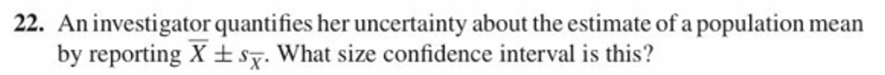# 22. An investigator quantifies her uncertainty about the estimate of a population meanby reporting X tsx. What size confidence interval is this?

Question
9 viewshelp_outlineImage Transcriptionclose22. An investigator quantifies her uncertainty about the estimate of a population mean by reporting X tsx. What size confidence interval is this? fullscreen
check_circle

Step 1

Confidence Interval:

Confidence interval is the interval estimate of the population parameter. It is the range where the population parameter value will lie in between.

The form of the confidence interval is,

CI = Point estimate ± Margin of error

The 100*(1–α)% confidence level means 100*(1–α)% of all possible sample values within the confidence interval will have the population parameter value and 100*α%  of sample values within the confidence interval will not have the population parameter.

The approximate 100*(1–α)% confidence interval for the population mean will be obtained using the formula given below:

Step 2

Central limit theorem:

If the sample size is large, then the sample mean will be approximately normally distributed with mean as population mean and standard deviation as (population standard deviation)/ SQRT(sample size), regardless of the distribution of the population from which the sample is taken.

In general the population standard deviation is rarely known. Hence, in practice sX-bar is used.

Therefore, the confidence interval will be defined as follows:

Step 3

Obtain the cumulative probability value:

The given confidence interval for population mean is X-bar ± sX-bar.

From the given confidence interval it is known that the critical value is zα/2 = 1.

Step-by-step procedure to obtain the cumulative probability value using the MINITAB software:

• Choose Calc > Probability Distributions.
• Choose ‘Normal’ distribution.
• Select Cumulative probability.
• Enter mean as 0 and standard deviation as
• Under...

### Want to see the full answer?

See Solution

#### Want to see this answer and more?

Solutions are written by subject experts who are available 24/7. Questions are typically answered within 1 hour.*

See Solution
*Response times may vary by subject and question.
Tagged in

### Statistics